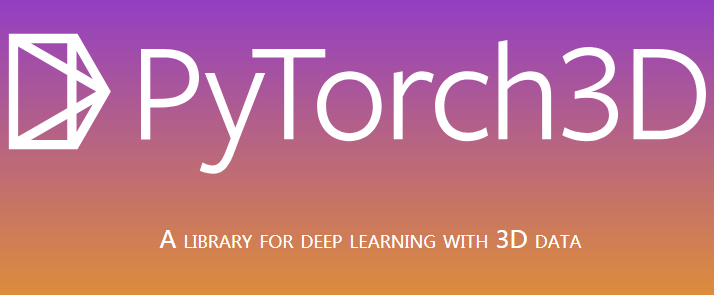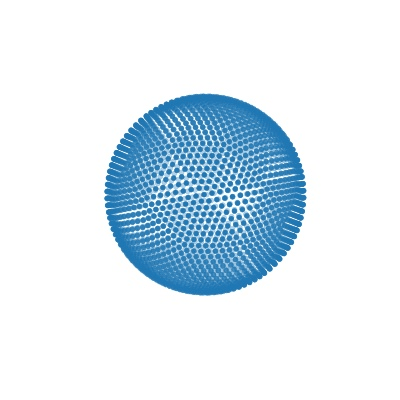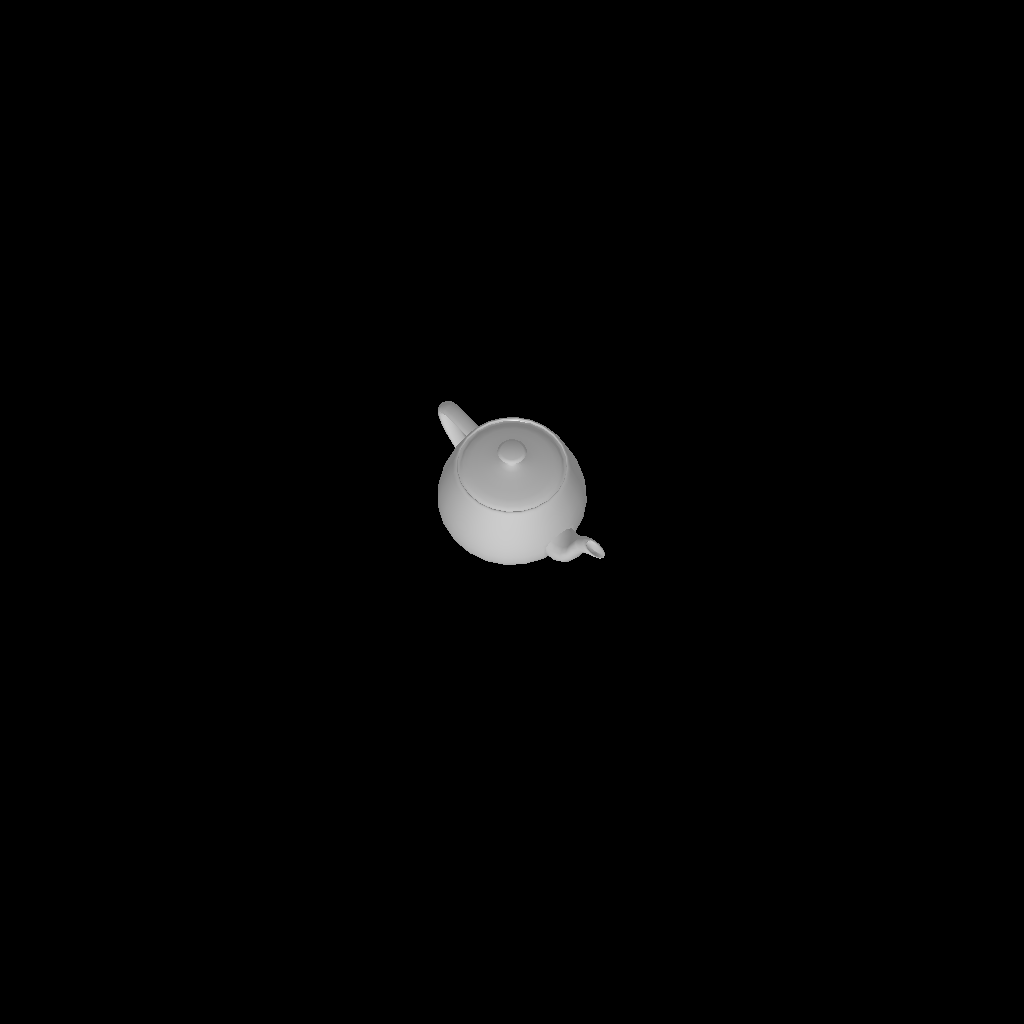# 3D 深度学习函数库PyTorch3D荐

BSD

2020-02-17

PyTorch3D 是一个用于处理 3D 数据的深度学习函数库，该库高度模块化且经过专门优化，具备独有的功能，旨在通过 PyTorch 简化 3D 深度学习。PyTorch3D 为 3D 数据提供了一组常用的 3D 运算符和快速且可微分的损失函数(loss function)，以及模块化的可微分渲染 API。PyTorch3D 主要特性

• 用于存储和操作三维物体的数据结构
• 可在三维物体上进行高效运算，诸如投影变换、图卷积、采样、损失函数等
• 可微分的三维物体渲染器

PyTorch3D 旨在与深度学习方法平稳集成，以预测和处理 3D 数据。因此，PyTorch3D 中的所有运算符：

• 使用 PyTorch 张量实现
• 可以对异构数据进行批量处理
• 可微分
• 可以利用 GPU 进行加速1. 安装 PyTorch3D
``````conda install pytorch torchvision -c pytorch  # OSX only
conda install pytorch3d -c pytorch3d  # all systems
``````
2. 尝试使用一些 3D 运算符，如计算两个网格之间的倒角损耗（ chamfer loss）
``````from pytorch3d.utils import ico_sphere
from pytorch3d.structures import Meshes
from pytorch3d.ops import sample_points_from_meshes
from pytorch3d.loss import chamfer_distance

# Use an ico_sphere mesh and load a mesh from an .obj e.g. model.obj
sphere_mesh = ico_sphere(level=3)
test_mesh = Meshes(verts=[verts], faces=[faces.verts_idx])

# Differentiably sample 5k points from the surface of each mesh and then compute the loss.
sample_sphere = sample_points_from_meshes(sphere_mesh, 5000)
sample_test = sample_points_from_meshes(test_mesh, 5000)
loss_chamfer, _ = chamfer_distance(sample_sphere, sample_test)``````

### Facebook 开源 3D 深度学习函数库 PyTorch3D，也可用于二维场景

02/17 08:15#### 暂无问答#### 暂无博客